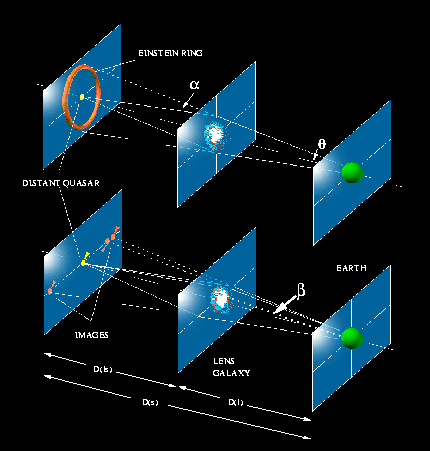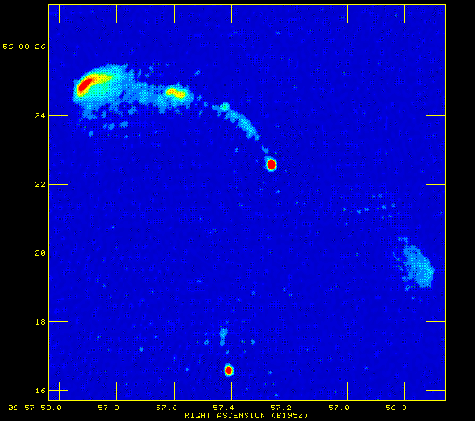# Introduction to lensingIllustration of a gravitational lens (courtesy A. Gunn).MERLIN image of the first known gravitational lens, 0957+561.

When a galaxy lies close to the line of sight to a distant quasar, the paths of the quasar's light and radio rays are bent by the galaxy's gravitational field. This can produce intensified, multiple images of the quasar - a phenomenon known as gravitational lensing.

If the line of sight to the quasar passes exactly through the galaxy, the symmetry of the system results in the formation of an ``Einstein ring''. If the line of sight is slightly off-centre, this produces multiple point images, as in the case of 0957+561, the first lens system discovered.

On the right is a MERLIN image of 0957+561. Note that the core of the quasar (the bright red point) has a double image, the secondary being about six arcseconds south of the primary image. Observations with optical telescopes show that these two images have the same optical spectrum, and so we can deduce that they are images of the same background object.

About 150-200 gravitational lens systems are now known. In some cases the background object is a quasar, and in some cases it is a galaxy. 22 of these were discovered by the CLASS survey, based at Jodrell Bank.

Apart from their intrinsic interest, gravitational lenses have exciting cosmological applications. Study of the lensed images, together with knowledge of gravitational physics, allows us to study the mass distribution of the lensing galaxy. This is important because gravitational lensing is sensitive to all matter, whether it emits light or not, and so allows us to measure both normal light-emitting matter, and also dark matter which is thought to make up much of the mass of galaxies. We are beginning to be able to make more detailed studies of mass distributions in galaxies, and test the degree to which the matter in galaxies is distributed in smaller lumps, as predicted by large numerical simulations with Cold Dark Matter.

If the background quasar is variable, the time delay between variations of two different components in the image allows us to calculate the difference in the paths of the corresponding rays from the distant quasar. Given the distances (i.e. redshifts) of the galaxy and quasar, and assuming that we can obtain a good enough mass model for the lensing galaxy, we can work out the absolute scale of the system -- and hence the Hubble expansion constant (Ho) can be calculated.

Last updated Fri Apr 18 16:56:17 2008